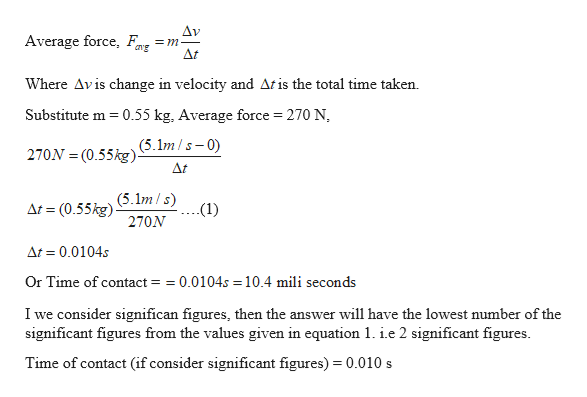A 0.55-kg croquet ball is initially at rest on the grass. When the ball is struck by a mallet, the average force exerted on it is 270 N.If the ball's speed after being struck is 5.1 m/s, for what amount of time was the mallet in contact with the ball? I solved this and got 0.0104 but mastering physics is saying that my answer is not correct.

Question

A 0.55-kg croquet ball is initially at rest on the grass. When the ball is struck by a mallet, the average force exerted on it is 270 N.

If the ball's speed after being struck is 5.1 m/s, for what amount of time was the mallet in contact with the ball?

I solved this and got 0.0104 but mastering physics is saying that my answer is not correct.

Step 1

Given:

Mass of the ball, m = 0.55 kg

Average Force on the ball, F = 270 N

Initial velocity of the ball, u = 0

Velocity of the ball after hit, v = 5.1 m/s

Step 2

Calculating the tim...help_outlineImage TranscriptioncloseΔν =m _ At Average force, g Where Avis change in velocity and Atis the total time taken Substitute m 0.55 kg, Average force = 270 N (5.1m/s-0 270N (0.55kg) At (5.1m/s) At (0.55kg) .(1) 270N At 0.0104s Or Time of contact = =0.0104s 10.4 mili seconds I we consider significan figures, then the answer will have the lowest number of the significant figures from the values given in equation 1. ie 2 significant figures Time of contact (if consider significant figures) 0.010 s fullscreen

Want to see the full answer?

See Solution

Want to see this answer and more?

Our solutions are written by experts, many with advanced degrees, and available 24/7

See Solution
Tagged in

Newtons Laws of Motion